### IMO Shortlist 1997 problem 3

Kvaliteta:
Avg: 0,0
Težina:
Avg: 0,0
For each finite set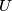$U$ of nonzero vectors in the plane we define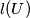$l(U)$ to be the length of the vector that is the sum of all vectors in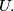$U.$ Given a finite set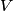$V$ of nonzero vectors in the plane, a subset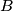$B$ of$V$ is said to be maximal if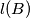$l(B)$ is greater than or equal to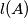$l(A)$ for each nonempty subset$A$ of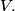$V.$

(a) Construct sets of 4 and 5 vectors that have 8 and 10 maximal subsets respectively.

(b) Show that, for any set$V$ consisting of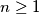$n \geq 1$ vectors the number of maximal subsets is less than or equal to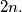$2n.$
Izvor: Međunarodna matematička olimpijada, shortlist 1997